# Commutative Property - Definition with Examples

The Complete K-5 Math Learning Program Built for Your Child

• 40 Million Kids

Loved by kids and parent worldwide

• 50,000 Schools

Trusted by teachers across schools

• Comprehensive Curriculum

Aligned to Common Core

## Commutative Property

The commutative property states that the numbers on which we operate can be moved or swapped from their position without making any difference to the answer. The property holds for Addition and Multiplication, but not for subtraction and division.

 Addition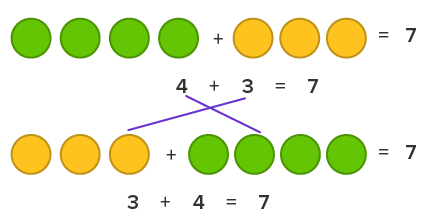Subtraction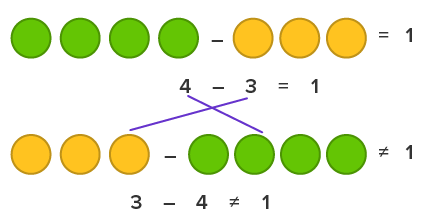MultiplicationDivision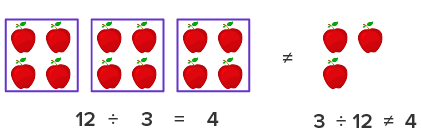The above examples clearly show that we can apply the commutative property on addition and multiplication. However, we cannot apply commutative property on subtraction and division. If you move the position of numbers in subtraction or division, it changes the entire problem.

Therefore, if a and b are two non-zero numbers, then:

The commutative property of addition is:

a + b = b + a

The commutative property of multiplication is:

a × b = b × a

In short, in commutative property, the numbers can be added or multiplied to each other in any order without changing the answer.

Let us see some examples to understand commutative property.

Example 1: Commutative property with addition

Myra has 5 marbles, and Rick has 3 marbles. How many marbles they have in total?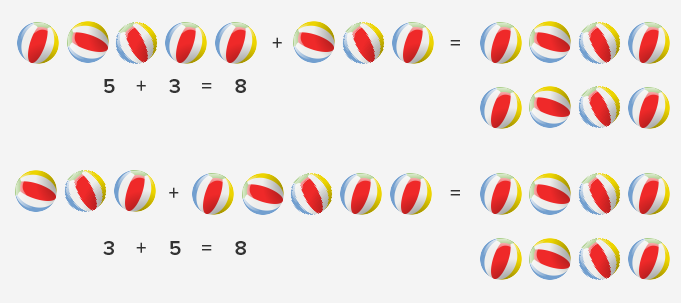Hence, we can see whether we add 5 + 3 or 3 + 5, the answer is always 8.

Example 2:  Commutative property with subtraction.

Alvin has 12 apples. He gives 8 apples to his sister. How many apples are left with Alvin?

Here, we subtract 8 from 12 and get the answer as 4 apples. However, we cannot subtract 12 from 8 and get 8 as the answer.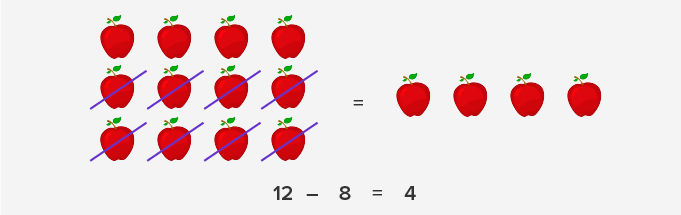Example 3: Commutative property with multiplication.

Sara buys 3 packs of buns. Each pack has 4 buns. How many buns did she buy?

Here, if we multiply 3 by 4 or 4 by 3, in both cases we get the answer as 12 buns.

So, the commutative property holds for multiplication.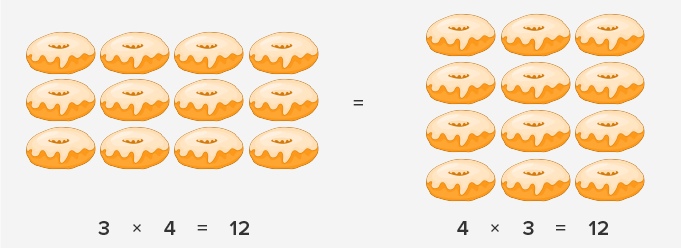So, commutative property holds true for multiplication.

Example 4: Commutative property with division.

If you have to divide 25 strawberries to 5 kids, each kid will receive 5 strawberries. However, if you have to divide 5 strawberries amongst 25 children, every kid will get a tiny fraction of the strawberry. Therefore, we cannot apply the commutative property with the division.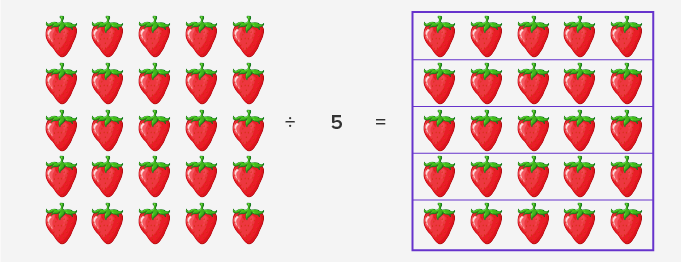Fun fact: Commutative property gets its name from the word commutes, meaning move around.

Won Numerous Awards & Honors